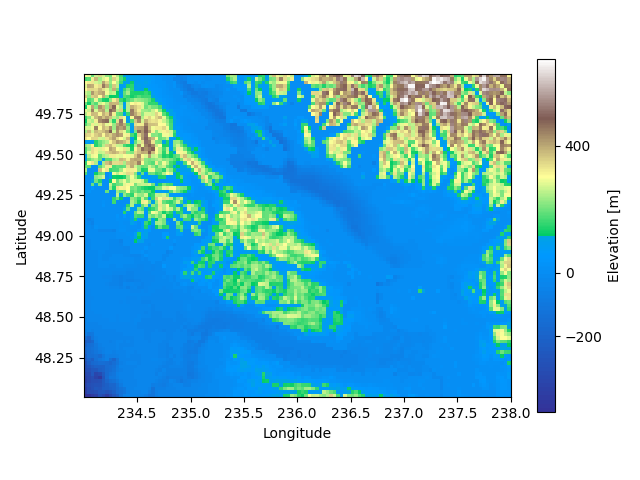# Pyplot: Different scales for normalized colorbars

Der Bei­trag die­se Woche ist gewis­ser­ma­ßen eine öffent­li­che Notiz für mich selbst.
Beim Erstel­len von Abbil­dun­gen für mei­ne Arbeit schaue ich oft die sel­ben Din­ge wie­der und wie­der nach. Des­halb habe ich mir gedacht, dass es sich viel­leicht lohnt, sol­che Din­ge hier zu sam­meln.
Schö­ner Neben­ef­fekt: wenn mich jemand fragt, wie etwas davon funk­tio­niert, kann ich ihn oder sie direkt hier hin schi­cken. Weil aller­dings die Leu­te, die mich sowas fra­gen, eher Eng­lisch als Deutsch spre­chen, wer­de ich die­se Art von Bei­trä­gen auf Eng­lisch schreiben.

Visua­li­zing two-dimen­sio­nal data sets can be qui­te hard, espe­cial­ly if not only qua­li­ta­ti­ve but also quan­ti­ta­ti­ve fea­tures should be clear­ly cap­tu­red. Often, a nice way around is to plot some kind of „heat­map“ visua­li­zing nume­ri­cal values at cer­tain points by dif­fe­rent colors. For examp­le, many topo­gra­phic maps indi­ca­ting the ele­va­ti­on do exact­ly this.

In many such cases, the­re is some rea­son­ab­le „cen­ter“ of the color­bar. For examp­le for a topo­gra­phic map indi­ca­ting ele­va­tions the sea level is often such a base­li­ne. Howe­ver, moun­tains often have a lar­ger ele­va­ti­on ran­ge than water has depth ran­ge. In such cases, it might be use­ful to use dif­fe­rent line­ar sca­les on both sides of the cen­ter value.

In this post, I’ll dis­cuss how to crea­te plots like the­se with Python’s Mat­plot­lib using code examp­les deri­ved from the Mat­plot­lib docu­men­ta­ti­on. In par­ti­cu­lar, I’ll com­ment on a weird ver­si­on-depen­dent beha­vi­or dif­fe­ring for Mat­plot­lib 3.4 and 3.5.

I tried to make this post as self con­tai­ned as pos­si­ble. The­re­fo­re, I first pre­sent the Python com­man­ds used step by step, while the com­ple­te code can be found here.

If you’­ve never used Mat­plot­lib befo­re, I recom­mend having a look at the who­le tool­kit – it’s real­ly ama­zing how simp­le it is to gene­ra­te nice figu­res with Matplotlib!

Befo­re visua­li­zing any data, we first have to load it. We’ll be using some examp­le data inclu­ded with the stan­dard Mat­plot­lib installation:

``````import matplotlib.cbook as cbook

# Compute elevations in meters instead of feet
topo = dem['topo']*0.3048

longitude = dem['longitude']
latitude = dem['latitude']``````

#### Creating the colormap

Next, we will crea­te our color­map based on the inclu­ded `terrain` colormap:

``````import numpy as np
import matplotlib.colors as colors
import matplotlib.pyplot as plt

colors_undersea = plt.cm.terrain(np.linspace(0, 0.17, 256))
colors_land = plt.cm.terrain(np.linspace(0.25, 1, 256))
all_colors = np.vstack((colors_undersea, colors_land))
terrain_map = colors.LinearSegmentedColormap.from_list('terrain_map', all_colors)``````

Based on this, we could now go ahead and plot our first topo­gra­phic map:

``````# Plot our map
pcm = plt.pcolormesh(longitude, latitude, topo, rasterized=True,

# Create a colorbar
cb = plt.colorbar(pcm, shrink=0.8)
cb.set_ticks([-200, 0, 400, 800, 1200])
cb.set_label('Elevation [m]')

# Set aspect ratio because distance between lines of longitude depends on latitude.

plt.show()``````First attempt for our topo­gra­phic map. Note the weird cen­ter of the color­bar – we’ll fix this in a second…

While this gives alrea­dy a nice first plot, note the weird posi­ti­on of the cen­ter of the color­bar. Right now, it is not cen­te­red at 0 m but some­whe­re around 50 m. This is defi­ni­te­ly not what we want, so we have to fix this.

#### Matplotlib’s TwoSlopeNorm

In fact, we can tell Mat­plot­lib to use dif­fe­rent line­ar sca­les on both sites of a cen­ter value using `TwoSlopeNorm`:

``````divnorm = colors.TwoSlopeNorm(vmin=-200., vcenter=0, vmax=1200.)

# Plot our map and tell Matplotlib to use our new normalized colormap
pcm = plt.pcolormesh(longitude, latitude, topo, rasterized=True,
Until now I could­n’t figu­re out a way to obtain the (in my opi­ni­on much nicer) con­sis­tent sca­le for posi­ti­ve and nega­ti­ve values using Mat­plot­lib 3.5.2. Luck­i­ly, you can sim­ply get the older ver­si­on using `pip install matplotlib==3.4.3` befo­re genera­ting your two-slo­ped color­map and updating again after­wards `pip install -U matplotlib`.